Скачать презентацию Chapter 18 Direct Current Circuits Electric Circuits

• Количество слайдов: 44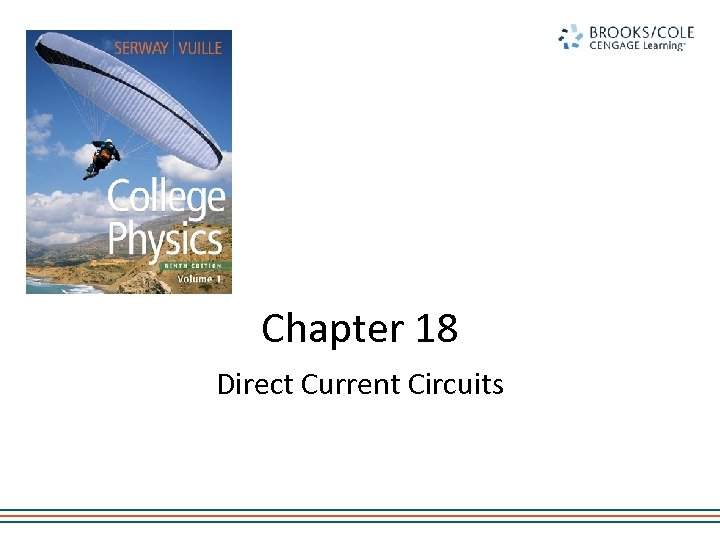Chapter 18 Direct Current Circuits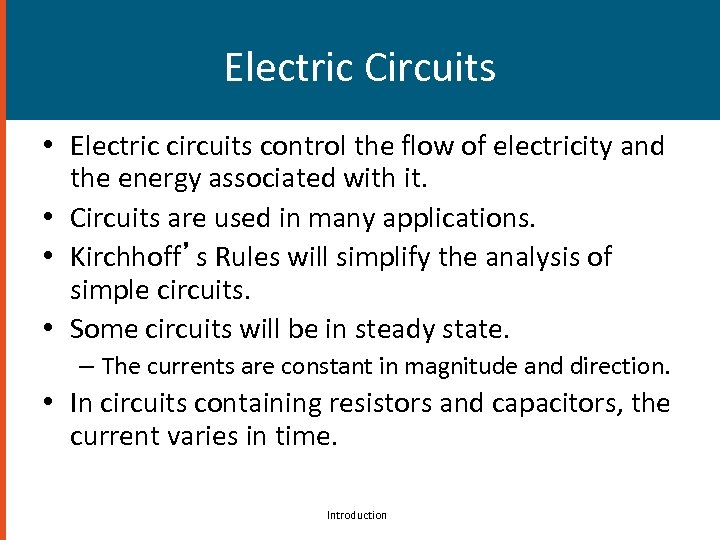Electric Circuits • Electric circuits control the flow of electricity and the energy associated with it. • Circuits are used in many applications. • Kirchhoff’s Rules will simplify the analysis of simple circuits. • Some circuits will be in steady state. – The currents are constant in magnitude and direction. • In circuits containing resistors and capacitors, the current varies in time. Introduction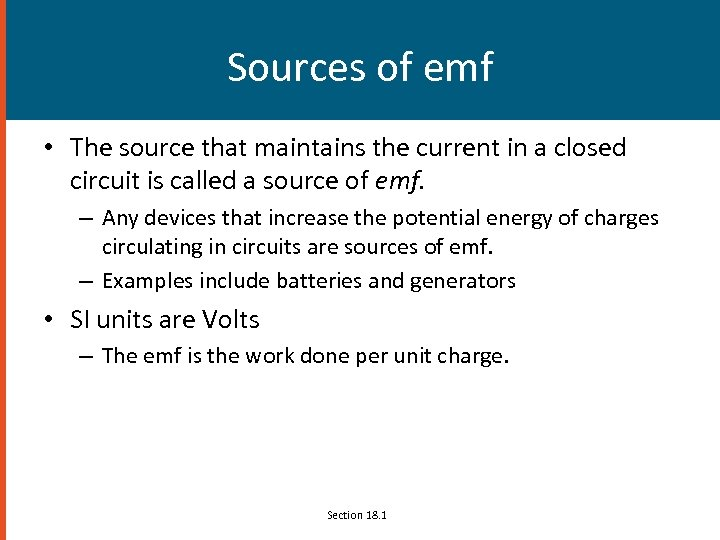Sources of emf • The source that maintains the current in a closed circuit is called a source of emf. – Any devices that increase the potential energy of charges circulating in circuits are sources of emf. – Examples include batteries and generators • SI units are Volts – The emf is the work done per unit charge. Section 18. 1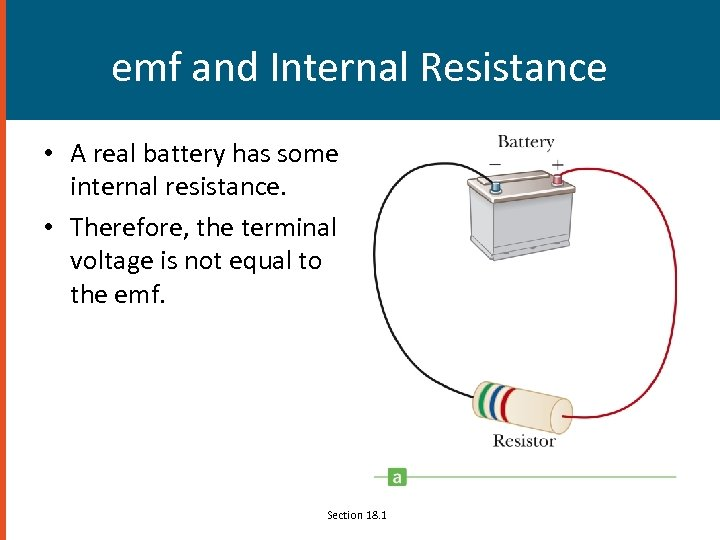emf and Internal Resistance • A real battery has some internal resistance. • Therefore, the terminal voltage is not equal to the emf. Section 18. 1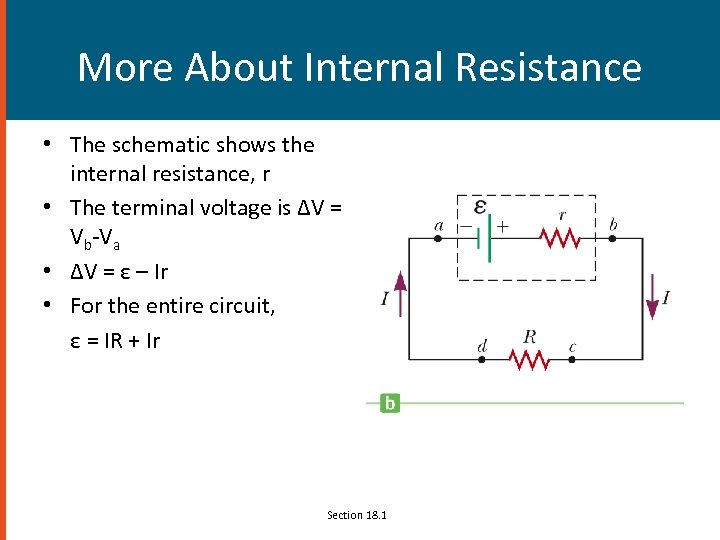More About Internal Resistance • The schematic shows the internal resistance, r • The terminal voltage is ΔV = Vb-Va • ΔV = ε – Ir • For the entire circuit, ε = IR + Ir Section 18. 1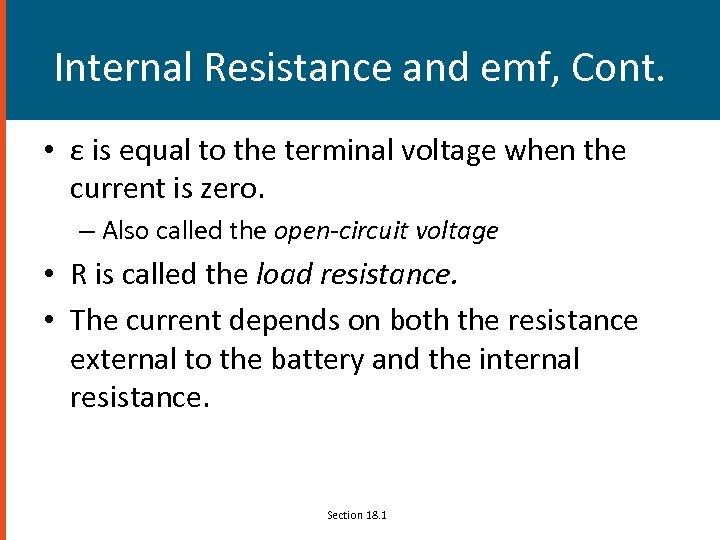Internal Resistance and emf, Cont. • ε is equal to the terminal voltage when the current is zero. – Also called the open-circuit voltage • R is called the load resistance. • The current depends on both the resistance external to the battery and the internal resistance. Section 18. 1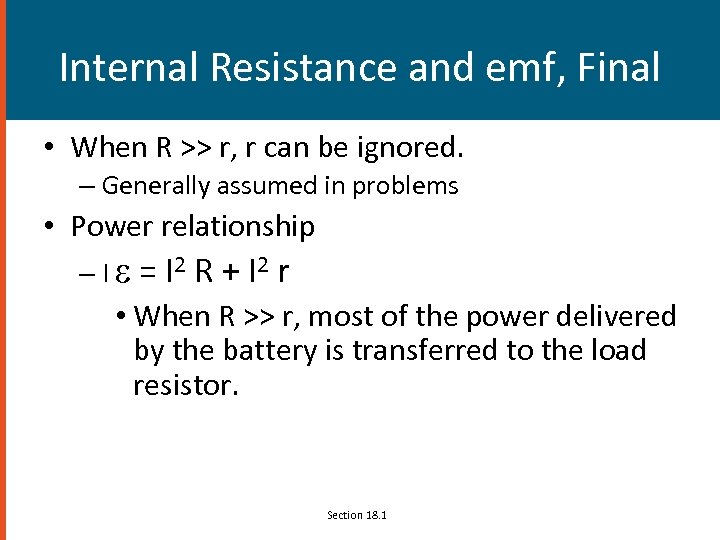Internal Resistance and emf, Final • When R >> r, r can be ignored. – Generally assumed in problems • Power relationship – I e = I 2 R + I 2 r • When R >> r, most of the power delivered by the battery is transferred to the load resistor. Section 18. 1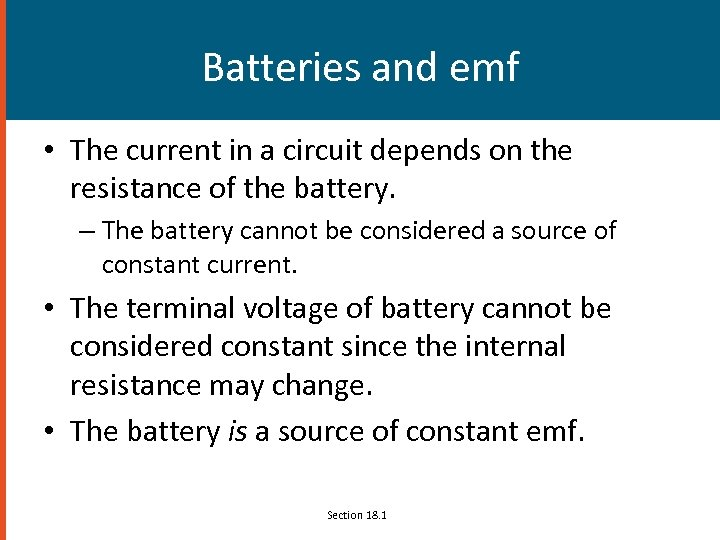Batteries and emf • The current in a circuit depends on the resistance of the battery. – The battery cannot be considered a source of constant current. • The terminal voltage of battery cannot be considered constant since the internal resistance may change. • The battery is a source of constant emf. Section 18. 1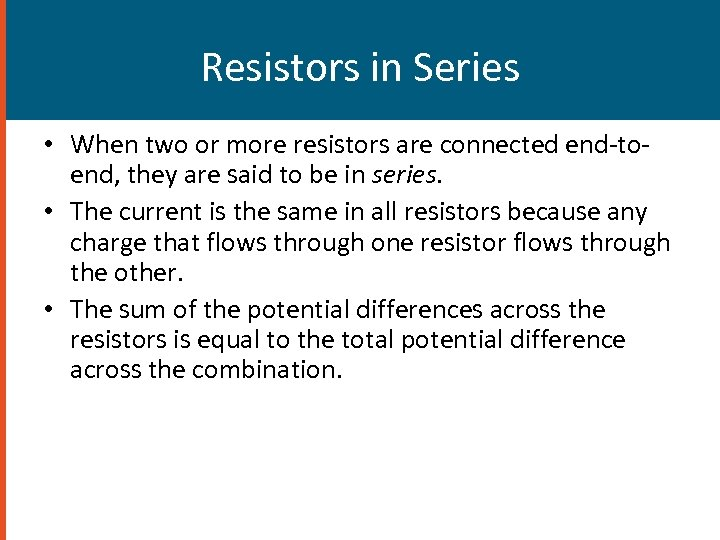Resistors in Series • When two or more resistors are connected end-toend, they are said to be in series. • The current is the same in all resistors because any charge that flows through one resistor flows through the other. • The sum of the potential differences across the resistors is equal to the total potential difference across the combination.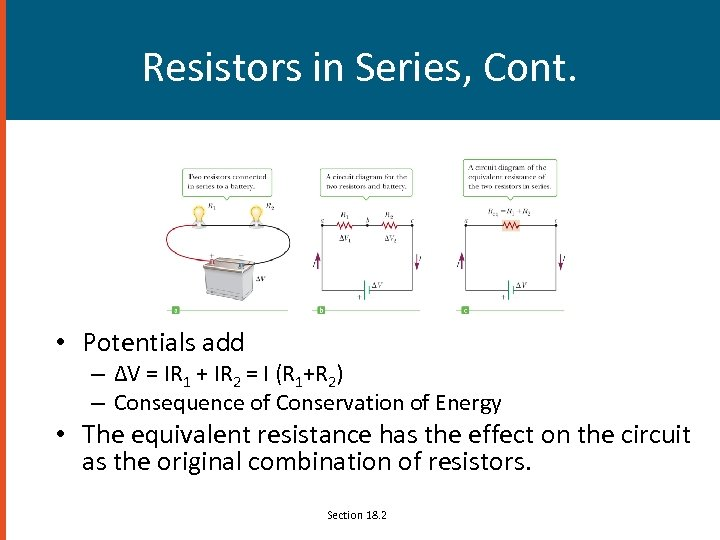Resistors in Series, Cont. • Potentials add – ΔV = IR 1 + IR 2 = I (R 1+R 2) – Consequence of Conservation of Energy • The equivalent resistance has the effect on the circuit as the original combination of resistors. Section 18. 2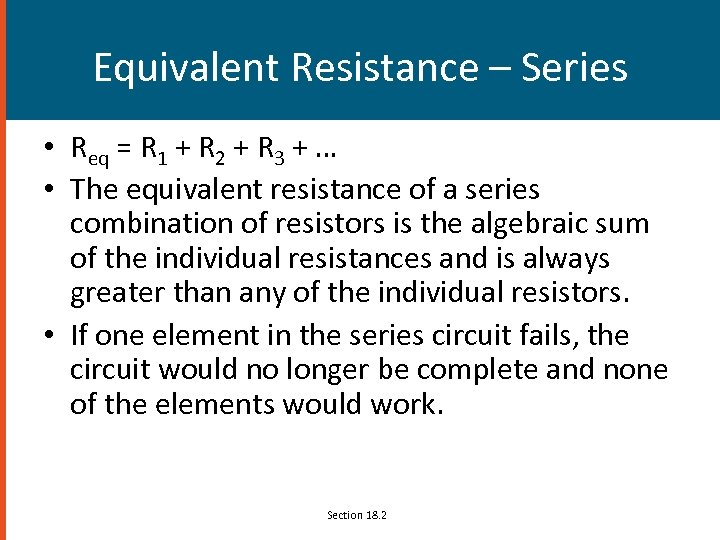Equivalent Resistance – Series • Req = R 1 + R 2 + R 3 + … • The equivalent resistance of a series combination of resistors is the algebraic sum of the individual resistances and is always greater than any of the individual resistors. • If one element in the series circuit fails, the circuit would no longer be complete and none of the elements would work. Section 18. 2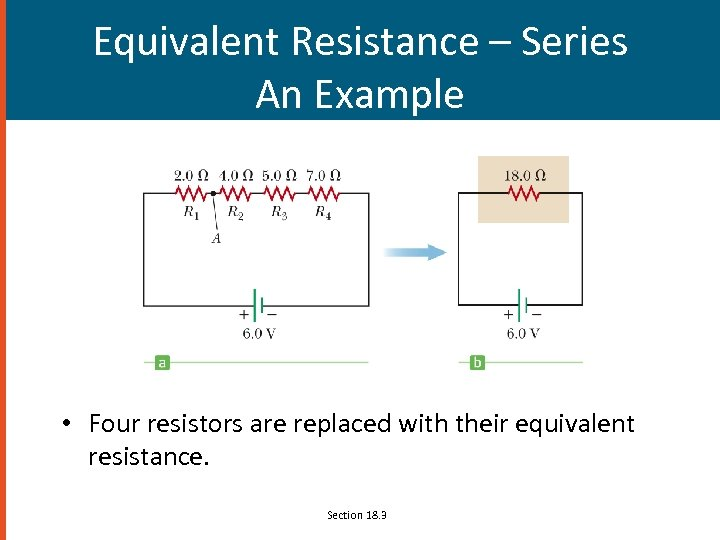Equivalent Resistance – Series An Example • Four resistors are replaced with their equivalent resistance. Section 18. 3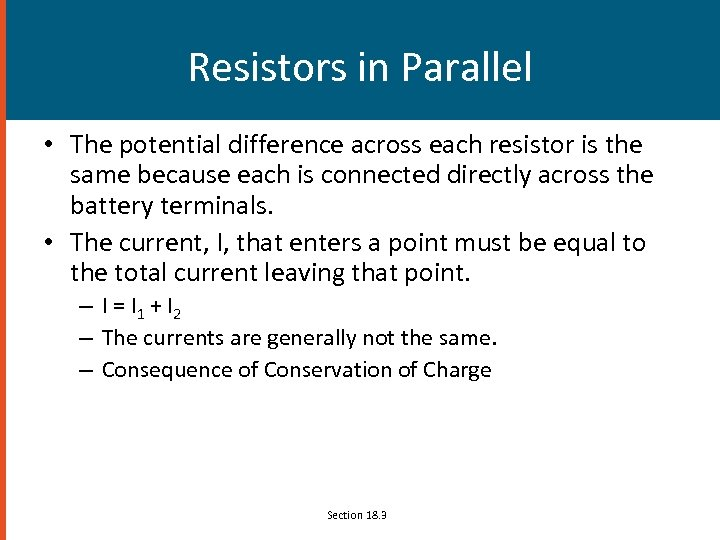Resistors in Parallel • The potential difference across each resistor is the same because each is connected directly across the battery terminals. • The current, I, that enters a point must be equal to the total current leaving that point. – I = I 1 + I 2 – The currents are generally not the same. – Consequence of Conservation of Charge Section 18. 3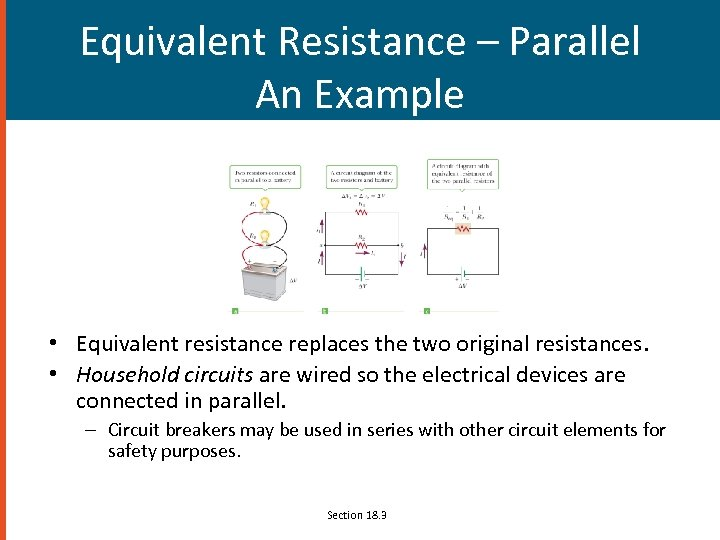Equivalent Resistance – Parallel An Example • Equivalent resistance replaces the two original resistances. • Household circuits are wired so the electrical devices are connected in parallel. – Circuit breakers may be used in series with other circuit elements for safety purposes. Section 18. 3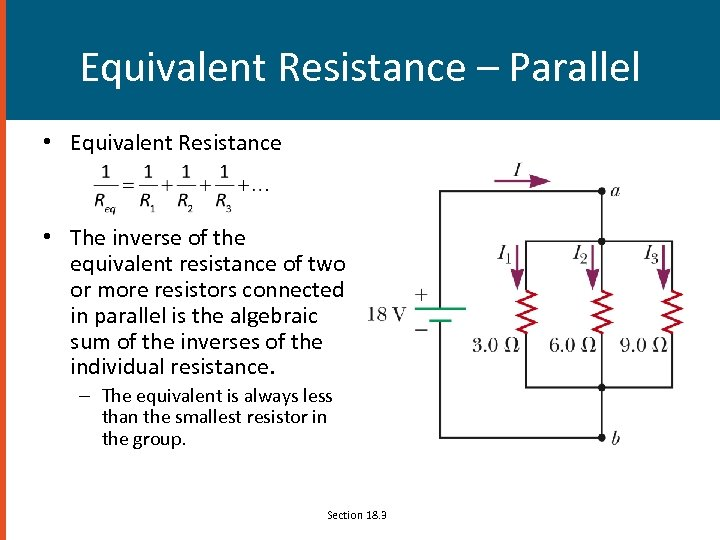Equivalent Resistance – Parallel • Equivalent Resistance • The inverse of the equivalent resistance of two or more resistors connected in parallel is the algebraic sum of the inverses of the individual resistance. – The equivalent is always less than the smallest resistor in the group. Section 18. 3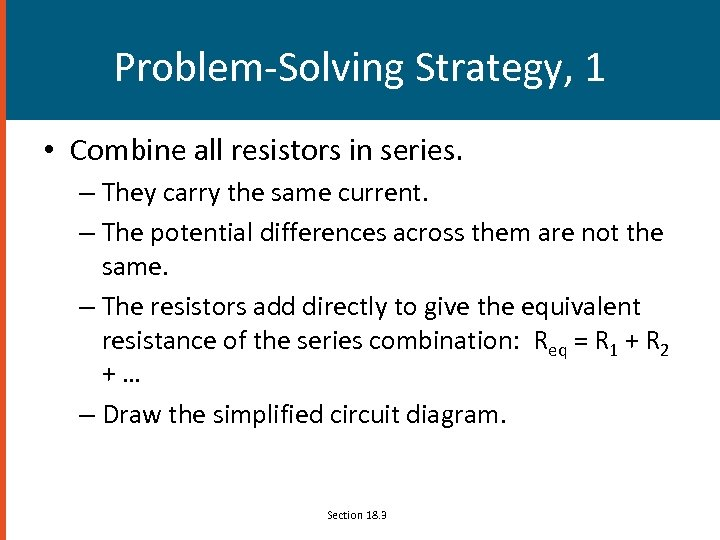Problem-Solving Strategy, 1 • Combine all resistors in series. – They carry the same current. – The potential differences across them are not the same. – The resistors add directly to give the equivalent resistance of the series combination: Req = R 1 + R 2 +… – Draw the simplified circuit diagram. Section 18. 3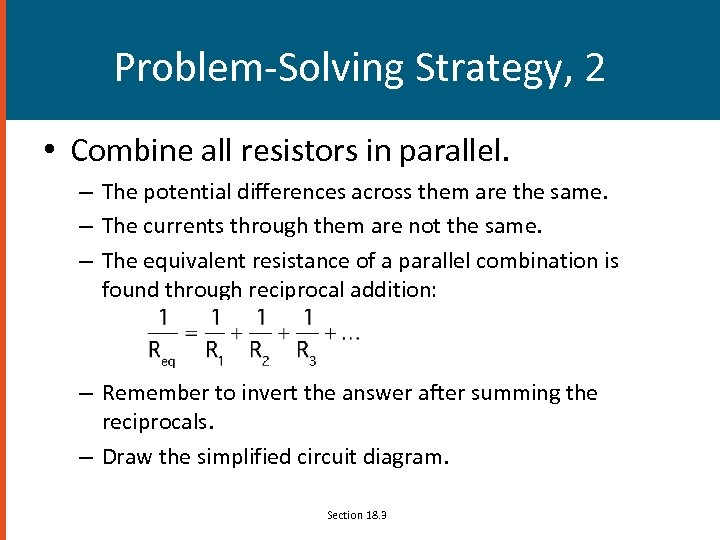Problem-Solving Strategy, 2 • Combine all resistors in parallel. – The potential differences across them are the same. – The currents through them are not the same. – The equivalent resistance of a parallel combination is found through reciprocal addition: – Remember to invert the answer after summing the reciprocals. – Draw the simplified circuit diagram. Section 18. 3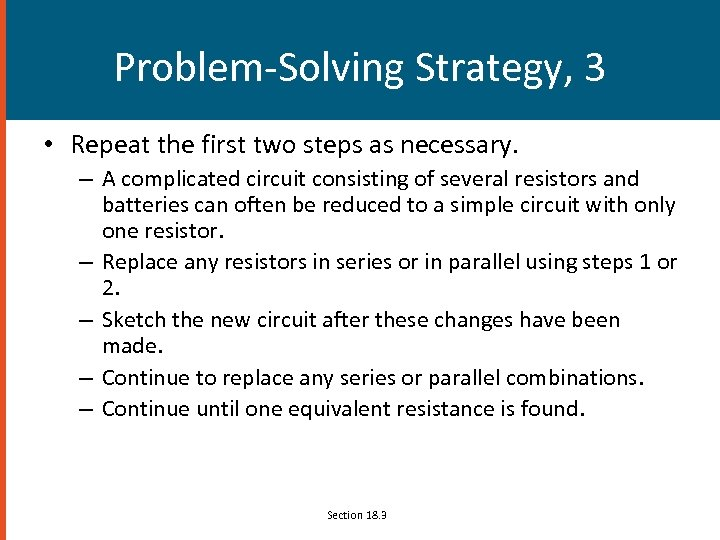Problem-Solving Strategy, 3 • Repeat the first two steps as necessary. – A complicated circuit consisting of several resistors and batteries can often be reduced to a simple circuit with only one resistor. – Replace any resistors in series or in parallel using steps 1 or 2. – Sketch the new circuit after these changes have been made. – Continue to replace any series or parallel combinations. – Continue until one equivalent resistance is found. Section 18. 3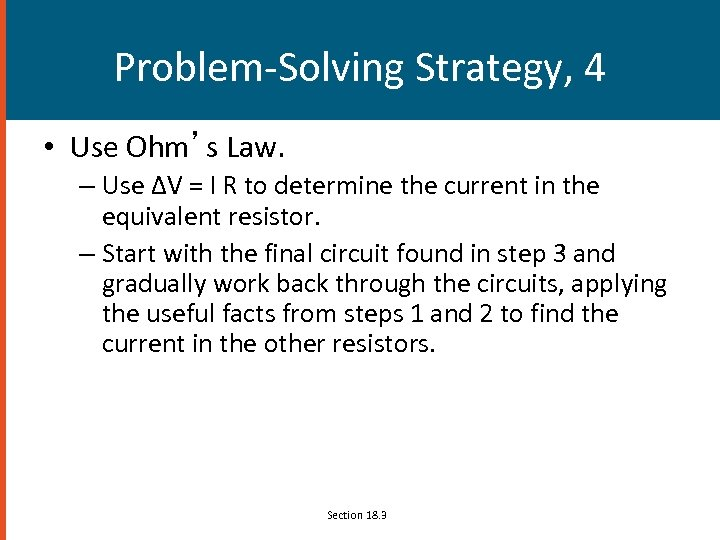Problem-Solving Strategy, 4 • Use Ohm’s Law. – Use ΔV = I R to determine the current in the equivalent resistor. – Start with the final circuit found in step 3 and gradually work back through the circuits, applying the useful facts from steps 1 and 2 to find the current in the other resistors. Section 18. 3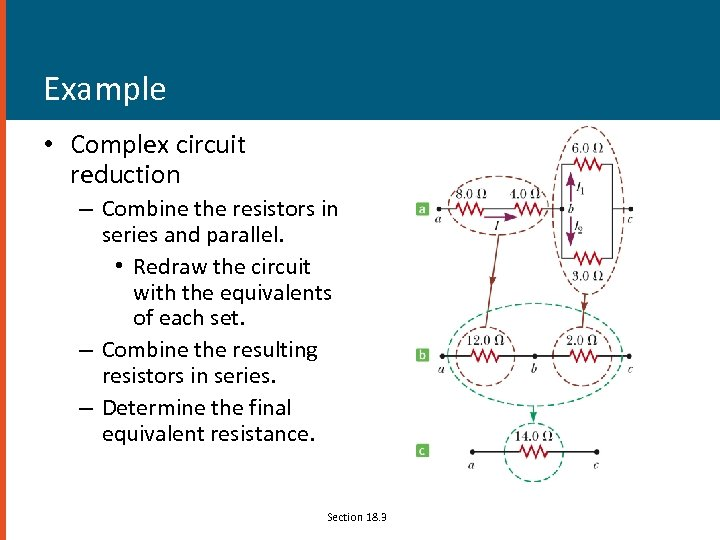Example • Complex circuit reduction – Combine the resistors in series and parallel. • Redraw the circuit with the equivalents of each set. – Combine the resulting resistors in series. – Determine the final equivalent resistance. Section 18. 3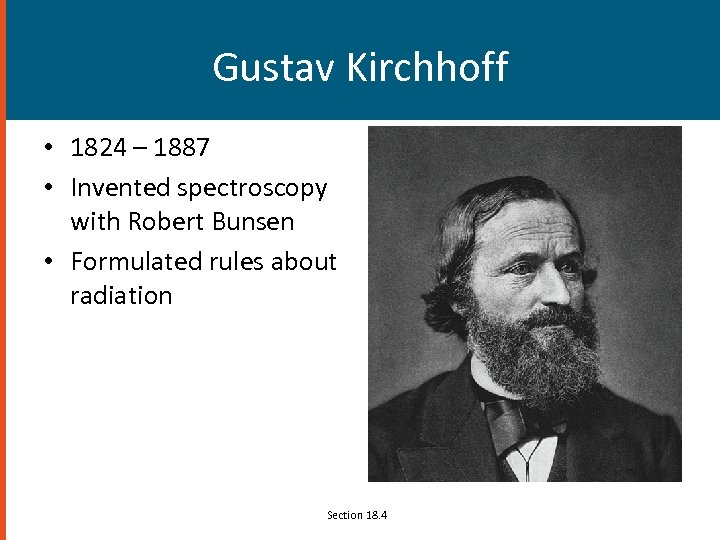Gustav Kirchhoff • 1824 – 1887 • Invented spectroscopy with Robert Bunsen • Formulated rules about radiation Section 18. 4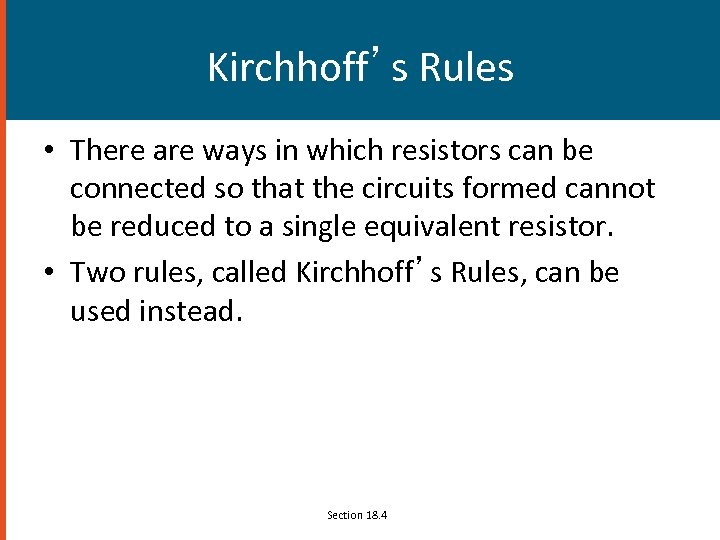Kirchhoff’s Rules • There are ways in which resistors can be connected so that the circuits formed cannot be reduced to a single equivalent resistor. • Two rules, called Kirchhoff’s Rules, can be used instead. Section 18. 4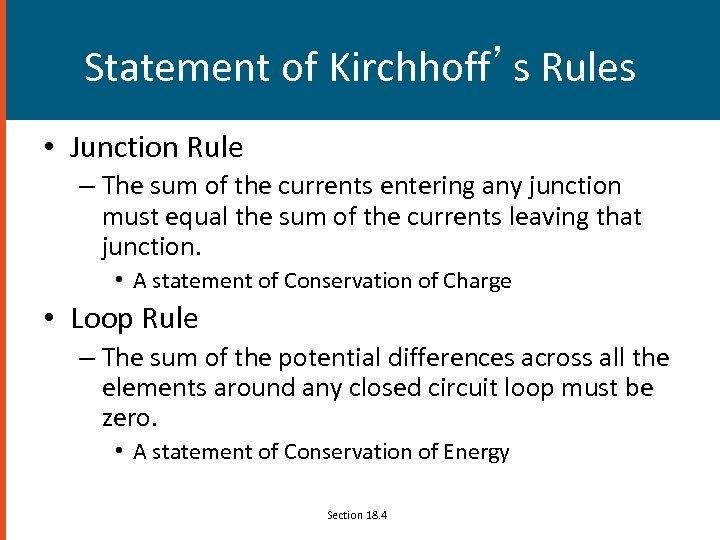Statement of Kirchhoff’s Rules • Junction Rule – The sum of the currents entering any junction must equal the sum of the currents leaving that junction. • A statement of Conservation of Charge • Loop Rule – The sum of the potential differences across all the elements around any closed circuit loop must be zero. • A statement of Conservation of Energy Section 18. 4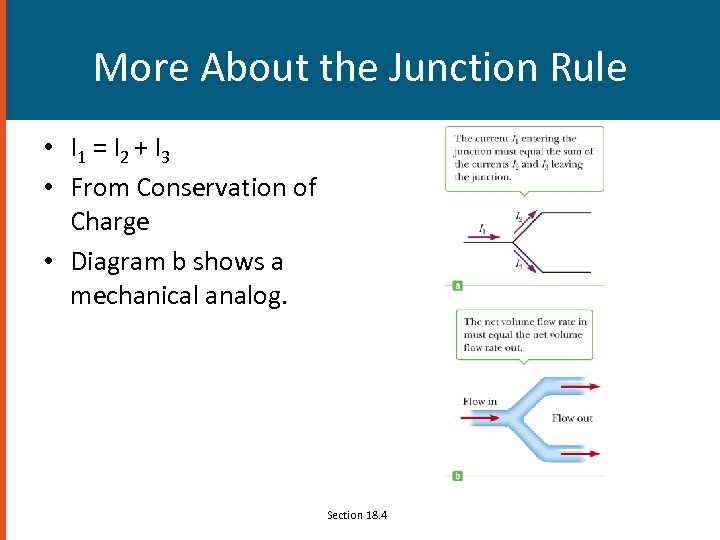More About the Junction Rule • I 1 = I 2 + I 3 • From Conservation of Charge • Diagram b shows a mechanical analog. Section 18. 4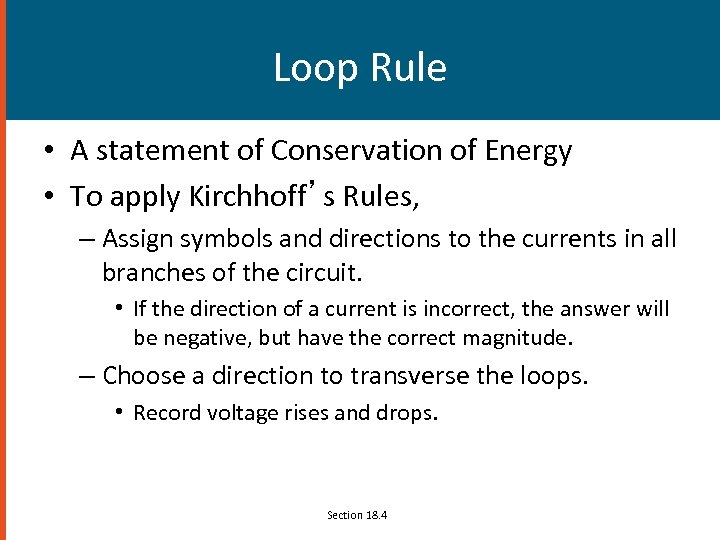Loop Rule • A statement of Conservation of Energy • To apply Kirchhoff’s Rules, – Assign symbols and directions to the currents in all branches of the circuit. • If the direction of a current is incorrect, the answer will be negative, but have the correct magnitude. – Choose a direction to transverse the loops. • Record voltage rises and drops. Section 18. 4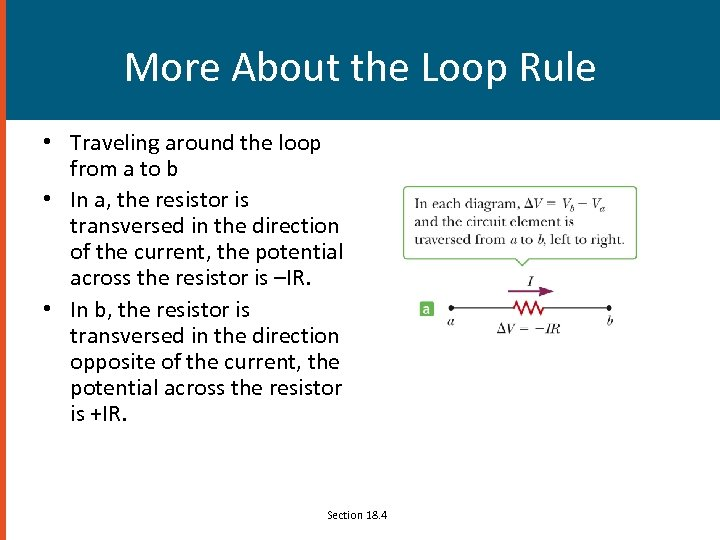More About the Loop Rule • Traveling around the loop from a to b • In a, the resistor is transversed in the direction of the current, the potential across the resistor is –IR. • In b, the resistor is transversed in the direction opposite of the current, the potential across the resistor is +IR. Section 18. 4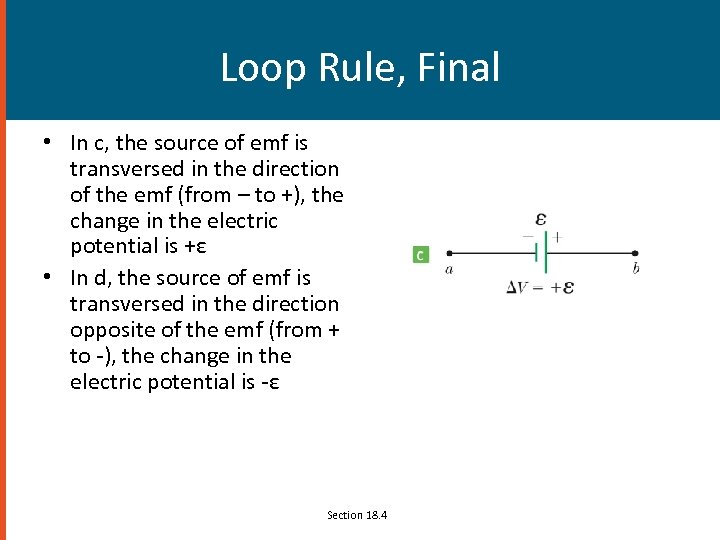Loop Rule, Final • In c, the source of emf is transversed in the direction of the emf (from – to +), the change in the electric potential is +ε • In d, the source of emf is transversed in the direction opposite of the emf (from + to -), the change in the electric potential is -ε Section 18. 4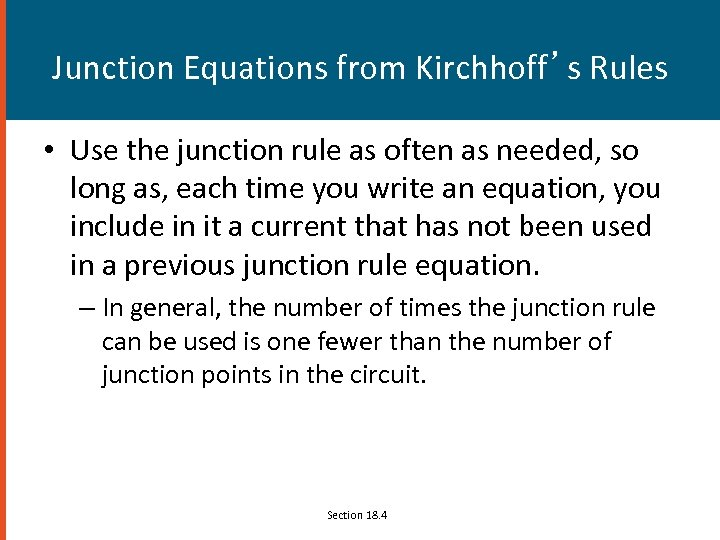Junction Equations from Kirchhoff’s Rules • Use the junction rule as often as needed, so long as, each time you write an equation, you include in it a current that has not been used in a previous junction rule equation. – In general, the number of times the junction rule can be used is one fewer than the number of junction points in the circuit. Section 18. 4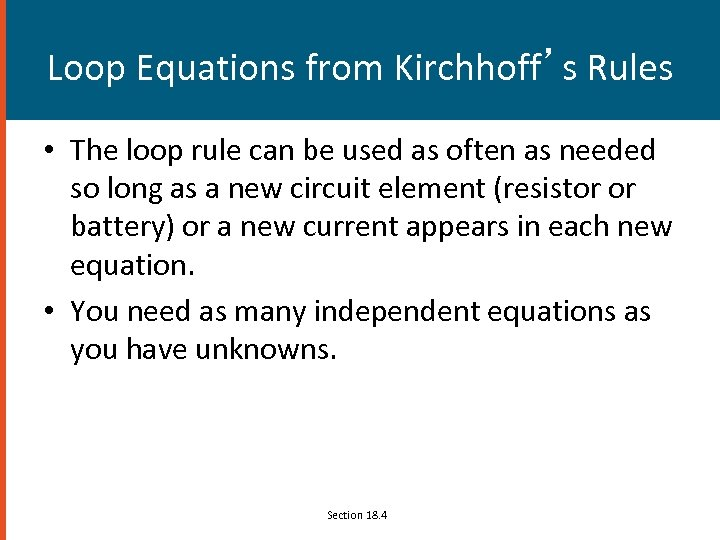Loop Equations from Kirchhoff’s Rules • The loop rule can be used as often as needed so long as a new circuit element (resistor or battery) or a new current appears in each new equation. • You need as many independent equations as you have unknowns. Section 18. 4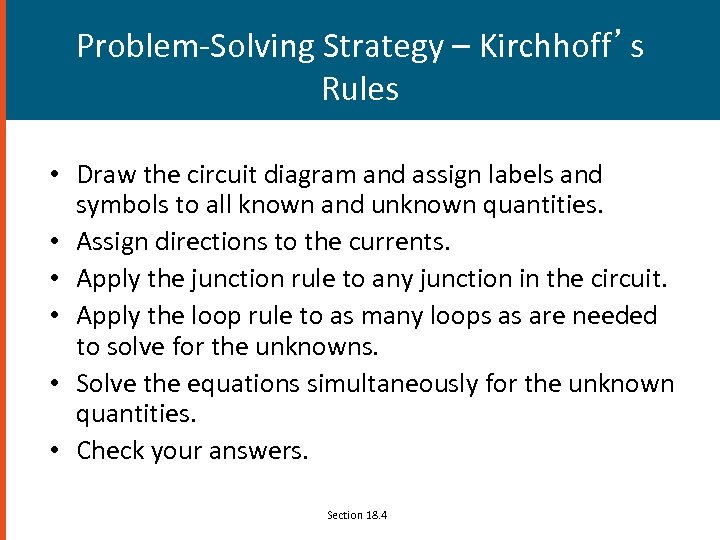Problem-Solving Strategy – Kirchhoff’s Rules • Draw the circuit diagram and assign labels and symbols to all known and unknown quantities. • Assign directions to the currents. • Apply the junction rule to any junction in the circuit. • Apply the loop rule to as many loops as are needed to solve for the unknowns. • Solve the equations simultaneously for the unknown quantities. • Check your answers. Section 18. 4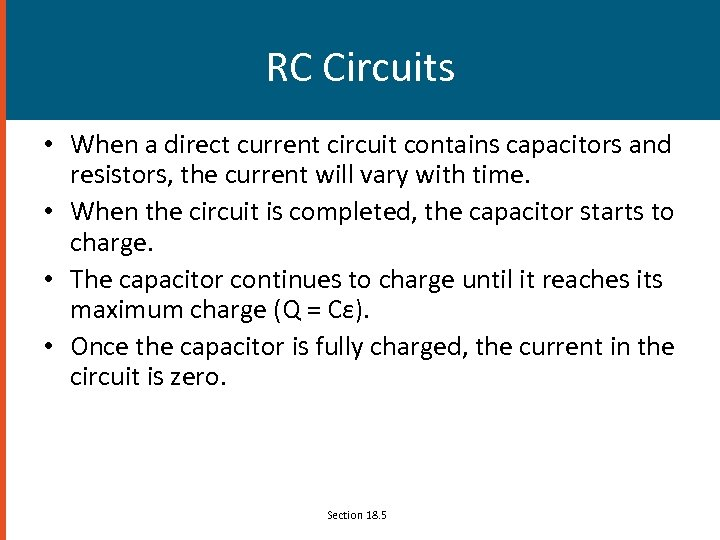RC Circuits • When a direct current circuit contains capacitors and resistors, the current will vary with time. • When the circuit is completed, the capacitor starts to charge. • The capacitor continues to charge until it reaches its maximum charge (Q = Cε). • Once the capacitor is fully charged, the current in the circuit is zero. Section 18. 5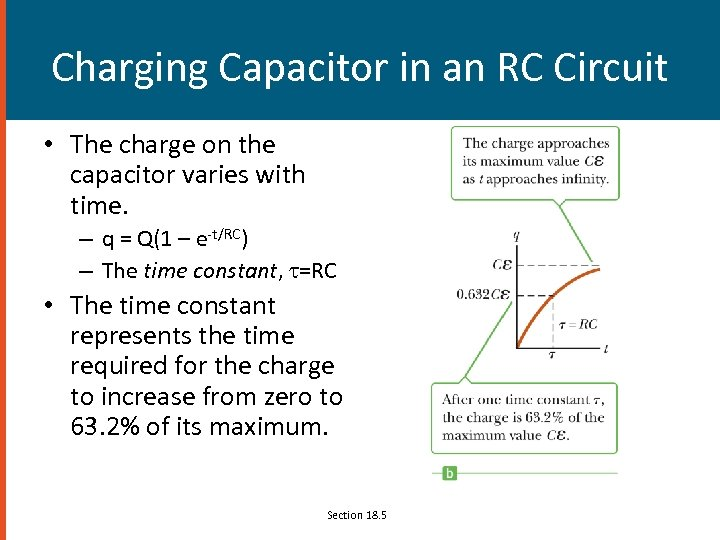Charging Capacitor in an RC Circuit • The charge on the capacitor varies with time. – q = Q(1 – e-t/RC) – The time constant, =RC • The time constant represents the time required for the charge to increase from zero to 63. 2% of its maximum. Section 18. 5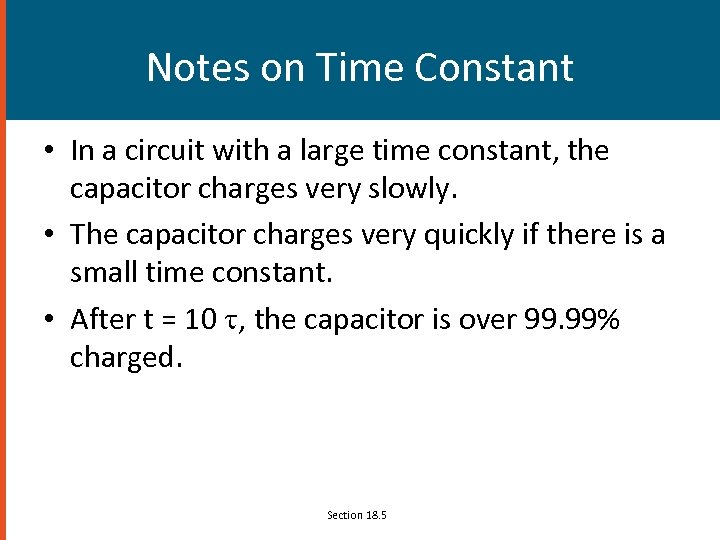Notes on Time Constant • In a circuit with a large time constant, the capacitor charges very slowly. • The capacitor charges very quickly if there is a small time constant. • After t = 10 , the capacitor is over 99. 99% charged. Section 18. 5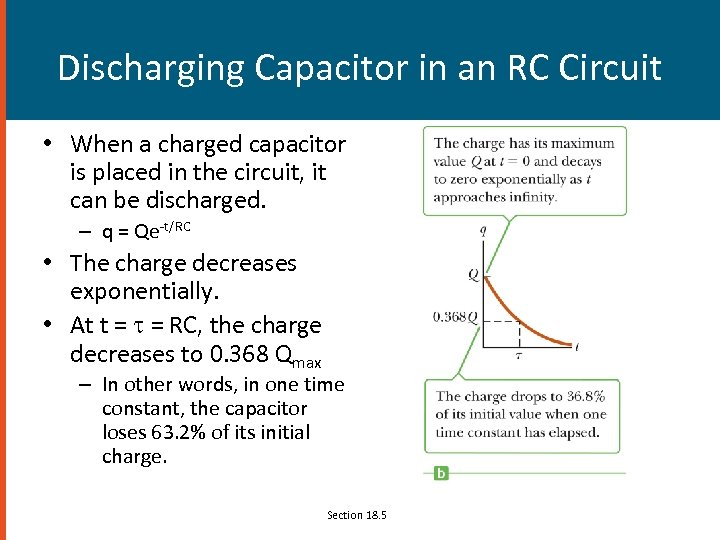Discharging Capacitor in an RC Circuit • When a charged capacitor is placed in the circuit, it can be discharged. – q = Qe-t/RC • The charge decreases exponentially. • At t = = RC, the charge decreases to 0. 368 Qmax – In other words, in one time constant, the capacitor loses 63. 2% of its initial charge. Section 18. 5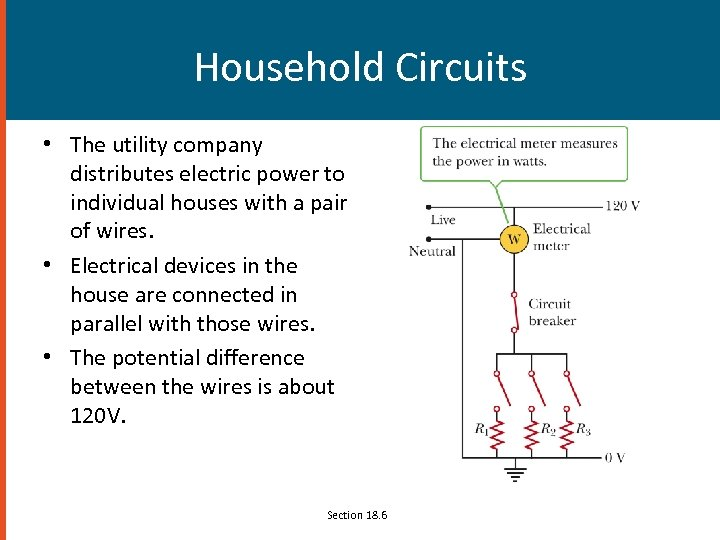Household Circuits • The utility company distributes electric power to individual houses with a pair of wires. • Electrical devices in the house are connected in parallel with those wires. • The potential difference between the wires is about 120 V. Section 18. 6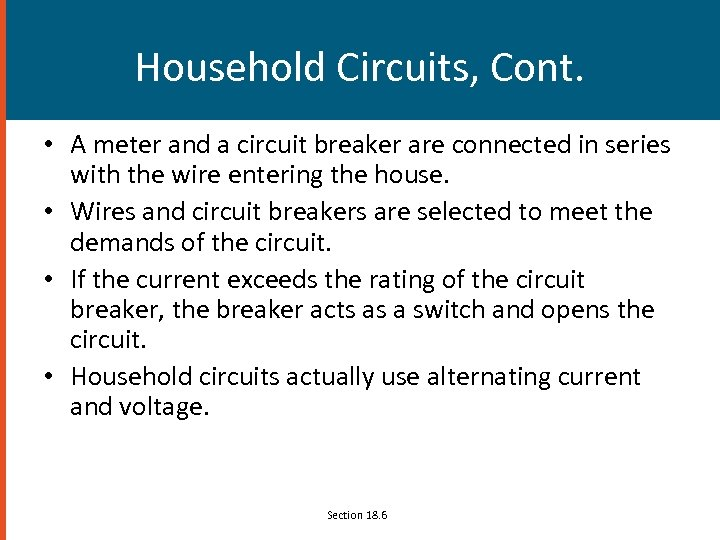Household Circuits, Cont. • A meter and a circuit breaker are connected in series with the wire entering the house. • Wires and circuit breakers are selected to meet the demands of the circuit. • If the current exceeds the rating of the circuit breaker, the breaker acts as a switch and opens the circuit. • Household circuits actually use alternating current and voltage. Section 18. 6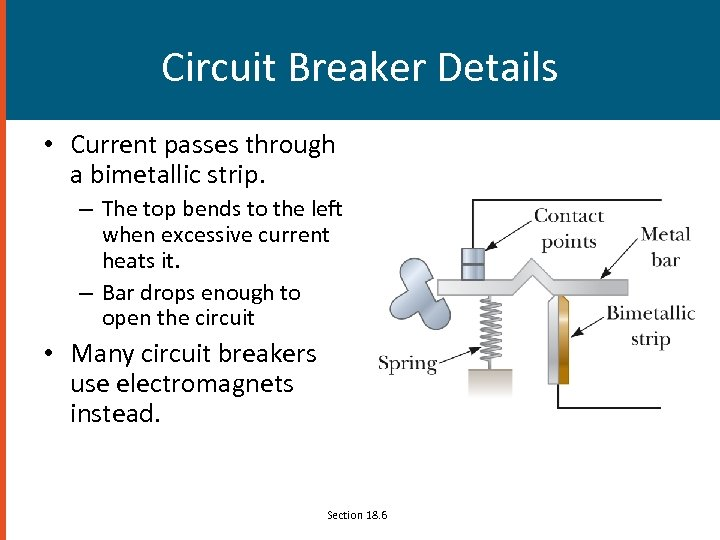Circuit Breaker Details • Current passes through a bimetallic strip. – The top bends to the left when excessive current heats it. – Bar drops enough to open the circuit • Many circuit breakers use electromagnets instead. Section 18. 6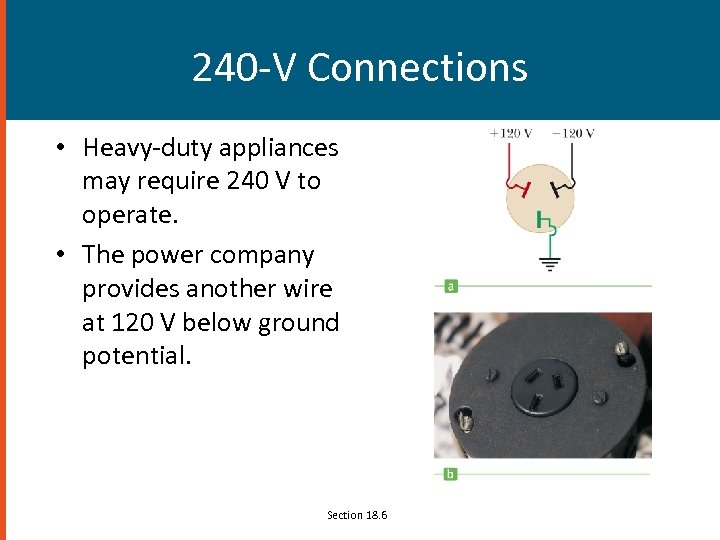240 -V Connections • Heavy-duty appliances may require 240 V to operate. • The power company provides another wire at 120 V below ground potential. Section 18. 6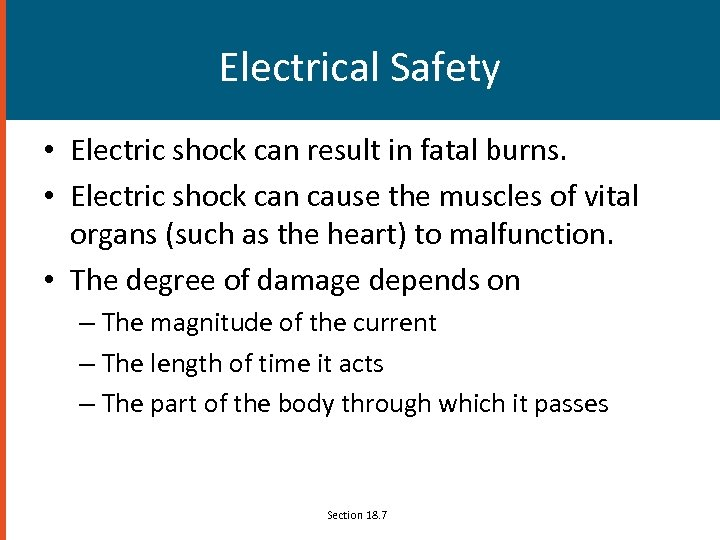Electrical Safety • Electric shock can result in fatal burns. • Electric shock can cause the muscles of vital organs (such as the heart) to malfunction. • The degree of damage depends on – The magnitude of the current – The length of time it acts – The part of the body through which it passes Section 18. 7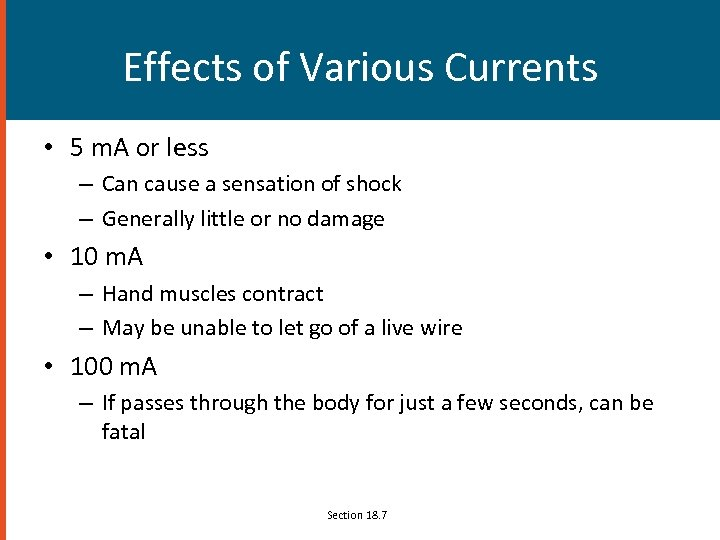Effects of Various Currents • 5 m. A or less – Can cause a sensation of shock – Generally little or no damage • 10 m. A – Hand muscles contract – May be unable to let go of a live wire • 100 m. A – If passes through the body for just a few seconds, can be fatal Section 18. 7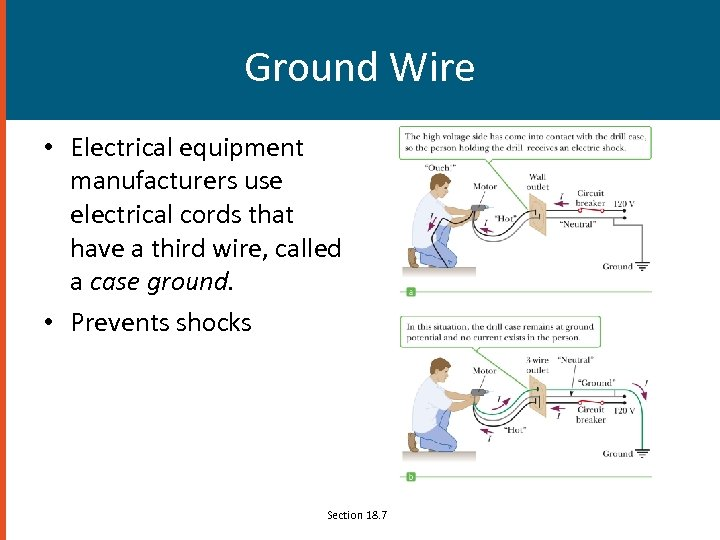Ground Wire • Electrical equipment manufacturers use electrical cords that have a third wire, called a case ground. • Prevents shocks Section 18. 7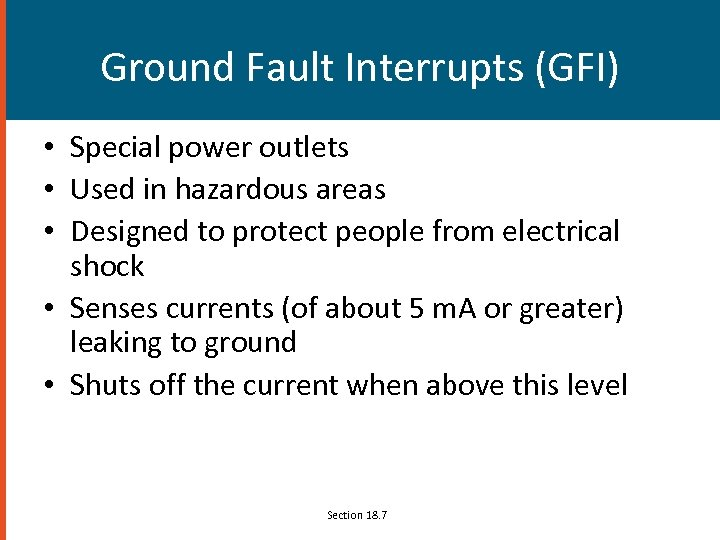Ground Fault Interrupts (GFI) • Special power outlets • Used in hazardous areas • Designed to protect people from electrical shock • Senses currents (of about 5 m. A or greater) leaking to ground • Shuts off the current when above this level Section 18. 7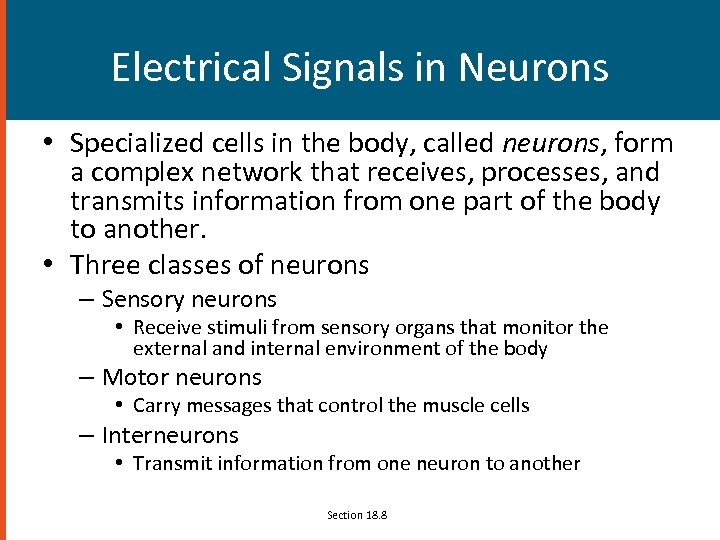Electrical Signals in Neurons • Specialized cells in the body, called neurons, form a complex network that receives, processes, and transmits information from one part of the body to another. • Three classes of neurons – Sensory neurons • Receive stimuli from sensory organs that monitor the external and internal environment of the body – Motor neurons • Carry messages that control the muscle cells – Interneurons • Transmit information from one neuron to another Section 18. 8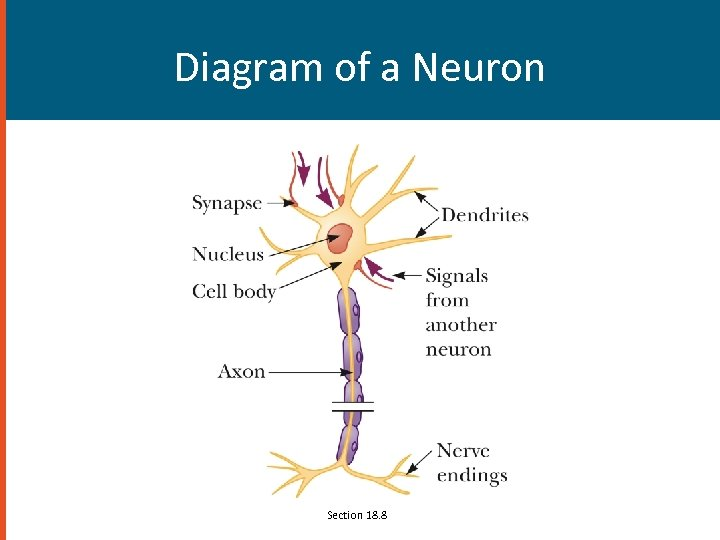Diagram of a Neuron Section 18. 8